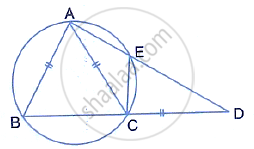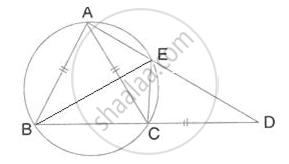Share

# In the Given Figure, Ab = Ac = Cd and ∠Adc = 38°. Calculate: (I) Angle Abc (Ii) Angle Bec - Mathematics

Course

#### Question

In the given figure, AB = AC = CD and ∠ADC = 38°. Calculate:
(i) Angle ABC
(ii) Angle BEC#### Solution(i) AC = CD
∴ ∠CAD = ∠CDA = 38°
∴∠ACD = 180° - 238° = 104°
∴∠ACB = 180° -104° = 76°             (Straight line)

Also, AB = AC
∴ ∠ABC = ACB = 76°

(ii) By angle sum property,
∠BAC = 180° - 2 ×76° = 38
∴ ∠BEC = ∠BAC = 38°
(Angles in the same chord)

Is there an error in this question or solution?